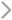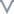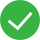DocumentationElastic Block Storage (EBS)User GuideTest the performance of an EBS volume

# Test the performance of an EBS volume

Last updated：2021-07-21 17:45:24

Test tool
We recommend that you use fio, the flexible I/O tester, to run a performance test on an EBS volume. You can run the following commands to install fio in the CentOS operating system:

``````sudo yum install libaio -y
sudo yum install libaio-devel -y
sudo yum install fio -y
``````

Parameter description

``````fio -filename=/dev/vdb -direct=1 -iodepth=32 -thread -rw=write -ioengine=libaio -bs=1m -size=200g -numjobs=1 -runtime=300 -group_reporting -name=test
``````
Parameter Description
-filename=/dev/vdb The name of the object to be tested. The parameter can be set to the name of a file, a raw disk, or a rados block device (RBD) image. In this example, it is set to the name of a raw disk. If the test object is a file system, set the parameter to a specific file name. If the test object is an RBD image, set the parameter to the name of a specific image. You can specify multiple objects at a time, in the format of -filename=/dev/vdb:/dev/vdc:/dev/vdd. Separate multiple objects with colons.
-direct=1 Specifies whether to use direct I/O and invalidate page cache of the operating system.
-iodepth=32 The depth of the I/O queue, namely the number of concurrent I/O requests sent to the system in a single thread. In synchronous mode, this parameter is set to 1. That is, only one I/O request is allowed in the queue. In asynchronous mode, you can set the parameter to a greater value so that the underlying I/O scheduling algorithms can be merged when multiple I/O requests are sent at a time. Generally, the parameter is set to 32 or 64 in asynchronous mode. Note that when you increase the number of allowed concurrent I/O requests, you need to consider the response time. As the queue depth increases, a single I/O request requires more time waiting in the queue, which prolongs the I/O response time. Make sure that you balance between the queue depth and response time. For the test with a single I/O request, set the parameter to 1. For the test with multiple I/O requests, set the parameter to 32.
-thread The mode of creating jobs. By default, fio creates multiple jobs, that is, multiple processes, by using the fork() function. If you use this parameter, fio creates multiple jobs by using the pthread_create() function.
-ioengine=libaio The way that the job issues I/O requests. Valid values: sync, psync, libaio, posixaio, rados, and rbd. The value sync indicates synchronous I/O by using the read() and write () functions. The value psync indicates synchronous I/O by using the pread() and pwrite () functions. Unlike the read() and write() functions, the pread() and pwrite () functions do not change the pointer when certain data is read from or written to the target file. The value libaio indicates Linux native asynchronous I/O. Linux only supports an asynchronous queue of non-buffered I/O. Therefore, the direct parameter must be set to 1. The value posixaio indicates POSIX asynchronous I/O. GNU C Library (Glibc) implements I/O delivery in the user space and users need to maintain multiple threads for asynchronous I/O requests. This mode consumes a large number of resources and lacks scalability. The value rados indicates a test on the I/O performance of the Reliable Autonomic Distributed Object Store (RADOS) layer by using the libaio API. The value rdb indicates a test on the I/O performance of the RBD image by using the librbd API. In this example, the libaio API is used to test the performance of the EBS volume.
-bs=1m The block size, which is the data size of each I/O request. If the EBS volume is used for a database, you can set the parameter to a small value, such as 4k or 8k. Consider the I/O operations per second (IOPS) when you set the parameter. If the EBS volume is used for the storage and archiving of objects with large sizes, such as videos, you can set the parameter to a large value, such as 1m or 4m. Consider the I/O throughput when you set the parameter. By default, to convert a large unit to a small unit whose symbols are written in lowercase letters, multiply the value with the large unit by 1,024. To convert a large unit to a small unit whose symbols are written in uppercase letters, multiply the value with the large unit by 1,000. Specifically, 1m = 1,024k and 1M = 1,000k. For a random read/write test, set the parameter to 4K. For a sequential read/write test, set the parameter to 1M.
-size=200g The total data size of I/O requests for the job. The parameter works together with the runtime parameter to control the running of fio. The fio tool stops if the actual data size or running duration reaches the corresponding parameter value that you specify. We recommend that you specify a large value for the parameter when you run a performance test, for example, 2g, 5g, 10g, or larger. We also recommend that you specify a value smaller than 4g for the parameter when you run a test on a file system.
-runtime=300 The running duration for the job. Unit: seconds. The parameter works together with the size parameter to control the running of fio. We recommend that you specify a large value for the parameter when you run a performance test, for example, 300 or 600.
-numjobs=1 The number of threads or processes tested concurrently. The thread parameter determines whether the threads or processes are tested. If you are running a performance test on an EBS volume, set the parameter to 1.
-group_reporting Specifies that the test results of all jobs are collected and summarized. If you do not use this parameter, test results are separate if multiple jobs are proceeding.
-name=test The name of the performance test.

Test results

``````1. iops: (g=0): rw=randwrite, bs=(R) 4096B-4096B, (W) 4096B-4096B, (T) 4096B-4096B, ioengine=libaio, iodepth=32
2. fio-3.1
3. Starting 1 process
4.
5. iops: (groupid=0, jobs=1): err= 0: pid=2476: Thu Apr  4 14:48:32 2019
6.   write: IOPS=19.9k, BW=78.1MiB/s (81.9MB/s)(22.9GiB/300004msec)
7.     slat (nsec): min=0, max=1275.7k, avg=4852.31, stdev=3764.04
8.     clat (usec): min=413, max=55302, avg=1592.61, stdev=6110.49
9.      lat (usec): min=446, max=55305, avg=1598.00, stdev=6110.56
10.     clat percentiles (usec):
11.      |  1.00th=[  611],  5.00th=[  660], 10.00th=[  685], 20.00th=[  717],
12.      |  30.00th=[  742],  40.00th=[  775], 50.00th=[  799], 60.00th=[  824],
13.      | 70.00th=[  857], 80.00th=[  898], 90.00th=[  971], 95.00th=[ 1057],
14.      |  99.00th=[  49546],  99.50th=[  50594], 99.90th=[  51119], 99.95th=[  51643],
15.      | 99.99th=
16.    bw (  KiB/s): min=47757, max=91609, per=99.97%, avg=79978.20, stdev=677.34, samples=3000
17.    iops        : min=11939, max=22902, avg=19994.52, stdev=169.35, samples=3000
18.   lat (usec)   : 500=0.01%, 750=31.87%, 1000=60.41%
19.   lat (msec)   : 2=5.97%, 4=0.12%, 10=0.03%, 20=0.01%, 50=0.71%
20.   lat (msec)   : 100=0.89%
21.   cpu          : usr=3.24%, sys=16.98%, ctx=1538323, majf=0, minf=86
22.   IO depths    : 1=0.1%, 2=0.1%, 4=0.1%, 8=0.1%, 16=0.1%, 32=100.0%, >=64=0.0%
23.      submit    : 0=0.0%, 4=100.0%, 8=0.0%, 16=0.0%, 32=0.0%, 64=0.0%, >=64=0.0%
24.      complete  : 0=0.0%, 4=100.0%, 8=0.0%, 16=0.0%, 32=0.1%, 64=0.0%, >=64=0.0%
25.      issued rwt: total=0,6000032,0, short=0,0,0, dropped=0,0,0
26.      latency   : target=0, window=0, percentile=100.00%, depth=32
27.
28. Run status group 0 (all jobs):
29.   WRITE: bw=78.1MiB/s (81.9MB/s), 78.1MiB/s-78.1MiB/s (81.9MB/s-81.9MB/s), io=22.9GiB (24.6GB), run=300004-300004msec
30.
32.  vdb: ios=61/5998000, merge=0/0, ticks=14/9424113, in_queue=9423702, util=100.00%
``````
Line No. Description
Line 1 The parameters used in the performance test by using fio.
Line 2 The version of fio used for the test.
Line 3 The number of fio jobs.
Line 6 The parameter values indicate the read/write mode, average IOPS, and average bandwidth, respectively. If you are running a random read/write test with the bs parameter set to 4k, pay attention to the average IOPS. If you are running a sequential read/write test with the bs parameter set to 1M, pay attention to the average bandwidth.
Line 7 The submission latency, that is, the time consumed for submitting an I/O request. The time starts from the time point when fio creates an I/O request to the time point when the kernel starts to process the request. This time is also referred to as the time waiting in the queue for execution. In synchronous I/O, this line is invisible, because no queue exists. The parameter values in this line indicate the minimum latency, maximum latency, average latency, and standard variance latency, respectively.
Line 8 The completion latency, that is, the time consumed for processing an I/O request. The time starts from the time point when the kernel starts to process an I/O request to the time point when the kernel completes the request. The time consumed for submitting the I/O request is excluded. The parameter values in this line indicate the minimum latency, maximum latency, average latency, and standard variance latency, respectively.
Line 9 The total response time, including slat and clat.
Line 10-15 The distribution of different percentages of I/O requests completed in the specified time.
Line 16 The minimum I/O bandwidth, maximum I/O bandwidth, percentage of aggregation I/O bandwidth occupied by a job, average I/O bandwidth, standard variance I/O bandwidth, and number of sampling points.
Line 17 The minimum IOPS, maximum IOPS, average IOPS, standard variance IOPS, and number of sampling points.
Line 18-20 The distribution of different percentages of I/O requests completed in the specified time. This parameter applies to all types of I/O requests.
Line 21 The information about CPU usage, including the CPU usage by the user, CPU usage by the kernel, number of context switches, number of major page faults, and number of minor page faults.
Line 22 The distribution of the I/O queue depth. The value of depth must be set to a power of two.
Line 23 The distribution of I/O submission, that is, the number of I/O requests submitted each time. In this example, the result 4=100% indicates that four I/O requests are submitted each time the submit() function is called.
Line 24 The distribution of I/O completion, that is, the number of I/O requests completed each time. In this example, the result 4=100% indicates that four I/O requests are completed each time. The result is affected by the I/O queue depth and I/O submission.
Line 28-29 The summary of the separate test results, applying to both reads and writes. The bw, io, and run parameters indicate the I/O bandwidth, total I/O data size, and running duration, respectively.
Line 31-32 The information about volume usage, including the volume name, total number of I/O requests, number of I/O requests merged by the I/O scheduler, number of ticks used for processing I/O requests, total time of I/O requests waiting in the queue for execution, and volume usage. The ios parameter indicates the total numbers of read requests and write requests, respectively. The merge parameter indicates the numbers of merged reads and merged writes, respectively. The ticks parameter indicates the numbers of ticks used for processing reads and writes, respectively.

References

Random write

``````fio -direct=1 -iodepth=1 -rw=randwrite -ioengine=libaio -bs=4k -size=1000G -numjobs=1 -runtime=300 -group_reporting -filename=/dev/vdb -name=Test_4K_RandWrite
``````

``````fio -direct=1 -iodepth=1 -rw=randread -ioengine=libaio -bs=4k -size=1000G -numjobs=1 -runtime=300 -group_reporting -filename=/dev/vdb -name=Test_4K_RandRead
``````

``````fio -direct=1 -iodepth=1 -rw=randrw -ioengine=libaio -bs=4k -size=1000G -numjobs=1 -runtime=300 -group_reporting -filename=/dev/vdb -name=Test_4K_RandRW
``````

Multi-way I/O
Random write

``````fio -direct=1 -iodepth=32 -rw=randwrite -ioengine=libaio -bs=4k -size=1000G -numjobs=1 -runtime=300 -group_reporting -filename=/dev/vdb -name=Test_4K_RandWrite
``````

``````fio -direct=1 -iodepth=32 -rw=randread -ioengine=libaio -bs=4k -size=1000G -numjobs=1 -runtime=300 -group_reporting -filename=/dev/vdb -name=Test_4K_RandRead
``````

``````fio -direct=1 -iodepth=32 -rw=randrw -ioengine=libaio -bs=4k -size=1000G -numjobs=1 -runtime=300 -group_reporting -filename=/dev/vdb -name=Test_4K_RandRW
``````

I/O throughput of sequential write

``````fio -direct=1 -iodepth=32 -rw=write -ioengine=libaio -bs=1m -size=1000G -numjobs=1 -runtime=300 -group_reporting -filename=/dev/vdb -name=Test_1m_ReqWrite
``````

``````fio -direct=1 -iodepth=32 -rw=read -ioengine=libaio -bs=1m -size=1000G -numjobs=1 -runtime=300 -group_reporting -filename=/dev/vdb -name=Test_1m_ReqRead
``````

``````fio -direct=1 -iodepth=32 -rw=rw -ioengine=libaio -bs=1m -size=1000G -numjobs=1 -runtime=300 -group_reporting -filename=/dev/vdb -name=Test_1m_ReqRW
``````

Did you find the above information helpful?UnhelpfulMostly UnhelpfulA little helpfulHelpfulVery helpful

#### What might be the problems?

Insufficient
Outdated
Unclear or awkward
Redundant or clumsy
Lack of context for the complex system or functionality

#### More suggestions

0/200